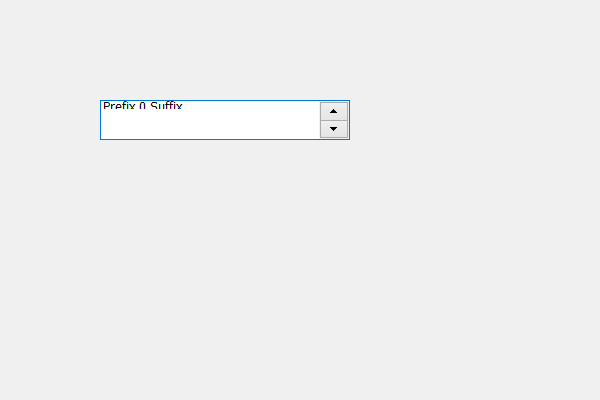GFG App
Open AppBrowser
Continue

# PyQt5 QSpinBox – Setting Bottom Margin

In this article we will see how we can set or change the bottom margin of the spin box, by default there is no margin set to the spin box i.e bottom margin value is zero. Although we can change that, setting margin to the spin box will not display any change in the spin box due to overriding take place by the widget to maintain its structure. But in order to set margin to the spin box we have set margin to the spin box’s line edit object by which we can display the margin in spin box. In order to do this we use setBottom method.

Syntax : margin.setBottom(n) Here margin is the QMargin object of the QLineEdit object which belongs to spin box Argument : It take integer as argument Return : It returns None

Implementation steps : 1. Create a spin box 2. Get the line edit object of the spin box 3. Get the margin object from the spin box 4. Set the bottom margin to the spin box 5. Add this margin object back to the line edit object Below is the implementation

## Python3

 `# importing libraries` `from` `PyQt5.QtWidgets ``import` `*` `from` `PyQt5 ``import` `QtCore, QtGui` `from` `PyQt5.QtGui ``import` `*` `from` `PyQt5.QtCore ``import` `*` `import` `sys`     `class` `Window(QMainWindow):`   `    ``def` `__init__(``self``):` `        ``super``().__init__()`   `        ``# setting title` `        ``self``.setWindowTitle("Python ")`   `        ``# setting geometry` `        ``self``.setGeometry(``100``, ``100``, ``600``, ``400``)`   `        ``# calling method` `        ``self``.UiComponents()`   `        ``# showing all the widgets` `        ``self``.show()`   `        ``# method for widgets` `    ``def` `UiComponents(``self``):` `        ``# creating spin box` `        ``self``.spin ``=` `QSpinBox(``self``)`   `        ``# setting geometry to spin box` `        ``self``.spin.setGeometry(``100``, ``100``, ``250``, ``40``)`   `        ``# setting range to the spin box` `        ``self``.spin.setRange(``0``, ``999999``)`   `        ``# setting prefix to spin` `        ``self``.spin.setPrefix("Prefix ")`   `        ``# setting suffix to spin` `        ``self``.spin.setSuffix(" Suffix")`   `        ``# getting line edit object` `        ``line_edit ``=` `self``.spin.lineEdit()`   `        ``# getting margins` `        ``margin ``=` `line_edit.contentsMargins()`   `        ``# setting bottom margin` `        ``margin.setBottom(``30``)`   `        ``# setting this margin back to line edit` `        ``line_edit.setContentsMargins(margin)`     `# create pyqt5 app` `App ``=` `QApplication(sys.argv)`   `# create the instance of our Window` `window ``=` `Window()` `window.spin.setFocus()` `# start the app` `sys.exit(App.``exec``())`

Output :My Personal Notes arrow_drop_up